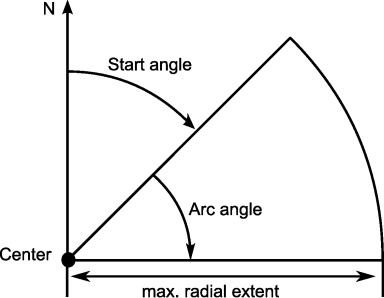2021.1.11

## Terrain Analysis EngineInterface ILcdLOSCoverage

• All Superinterfaces:
java.lang.Cloneable
All Known Subinterfaces:
ILcdLOSCoverageMatrix
All Known Implementing Classes:
TLcdLOSCoverage

```public interface ILcdLOSCoverage
extends java.lang.Cloneable```
Defines a circular area around a center point, used as input for line-of-sight computations. It represents the area in an arc, starting from a start angle and extending over an arc angle, and contained within a maximum radius. It also defines a radial and angular discretization step size. It requires the following information:
• The center point (`ILcdPoint`) around which the area is covered.
• The center point reference (`ILcdGeoReference`) in which the center point is defined.
• The altitude of the center point.
• The altitude mode in which the altitude of the center point should be interpreted..
• The maximum radial extent of the coverage, defined in meters.
• The radius step size to define a radial discretization, defined in meters.
• The start angle of the coverage, defined in degrees.
• The arc angle of the coverage, defined in degrees.
• The angle step size to define an angular discretization, defined in degrees.

These properties are explained further in the following image:• ### Method Summary

All Methods
Modifier and Type Method and Description
`java.lang.Object` `clone()`
Making the clone method available for all other classes.
`double` `getAngleArc()`
Returns the arc angle of this coverage, defined in degrees.
`double` `getAngleStart()`
Returns the start angle of this coverage, defined in degrees.
`double` `getAngleStep()`
Returns the angle step of this coverage, defined in degrees.
`ILcdPoint` `getCenterPoint()`
Returns the center point of this coverage.
`double` `getCenterPointAltitude()`
Returns the altitude of the center point, defined by the `getCenterPointAltitudeMode()`.
`TLcdCoverageAltitudeMode` `getCenterPointAltitudeMode()`
Returns the altitude mode in which the altitude of the center point should be interpreted.
`ILcdGeoReference` `getCenterPointReference()`
Returns the center point reference of this coverage.
`double` `getRadiusMax()`
Returns the maximum radial extent of this coverage, defined in meters.
`default double` `getRadiusSampleDistance(int aIndex)`
Returns the distance in meters from center at which sample with index aIndex was taken.
`double` `getRadiusStep()`
Returns the radius step of this coverage, defined in meters.
`default double` `getRadiusStep(int aIndex)`
Returns the radius step of this coverage, defined in meters, at the given index.
• ### Method Detail

• #### getAngleArc

`double getAngleArc()`
Returns the arc angle of this coverage, defined in degrees.
Returns:
the arc angle of this coverage, defined in degrees.
• #### getAngleStart

`double getAngleStart()`
Returns the start angle of this coverage, defined in degrees.
Returns:
the start angle of this coverage, defined in degrees.
• #### getAngleStep

`double getAngleStep()`
Returns the angle step of this coverage, defined in degrees.
Returns:
the angle step of this coverage, defined in degrees.
• #### getCenterPoint

`ILcdPoint getCenterPoint()`
Returns the center point of this coverage.
Returns:
the center point of this coverage.
• #### getCenterPointAltitudeMode

`TLcdCoverageAltitudeMode getCenterPointAltitudeMode()`
Returns the altitude mode in which the altitude of the center point should be interpreted.
Returns:
the altitude mode in which the altitude of the center point should be interpreted.
• #### getCenterPointReference

`ILcdGeoReference getCenterPointReference()`
Returns the center point reference of this coverage.
Returns:
the center point reference of this coverage.

`double getRadiusMax()`
Returns the maximum radial extent of this coverage, defined in meters.
Returns:
the maximum radial extent of this coverage, defined in meters.

`double getRadiusStep()`
Returns the radius step of this coverage, defined in meters. For coverages with nonuniform radius steps, use `getRadiusStep(int)`.
Returns:
the radius step of this coverage, defined in meters.
`getRadiusStep(int)`

`default double getRadiusStep(int aIndex)`
Returns the radius step of this coverage, defined in meters, at the given index. A step at index i refers to the step size between `radius sample distance` i and i + 1. If this coverage was created with regular, uniform sampling the radius step is the same for all indices.
Parameters:
`aIndex` - the index
Returns:
the radius step of this coverage, defined in meters, at the given index.
Since:
2018.1

`default double getRadiusSampleDistance(int aIndex)`
Returns the distance in meters from center at which sample with index aIndex was taken.
Parameters:
`aIndex` - the index
Returns:
distance in meters from center at which sample with index aIndex was taken.
Since:
2018.1
• #### clone

```java.lang.Object clone()
throws java.lang.CloneNotSupportedException```
Making the clone method available for all other classes. All implementations of this interface should support the clone method. This means that this method should never throw a CloneNotSupportedException.
Returns:
a clone of this instance.
Throws:
`java.lang.CloneNotSupportedException`